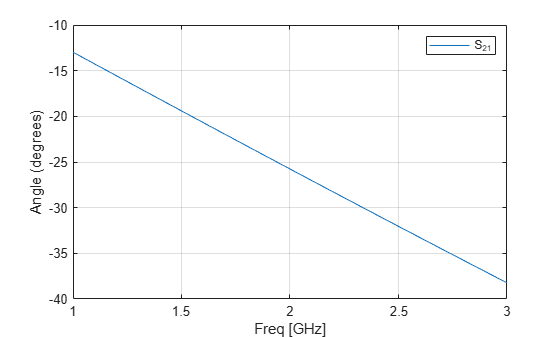Documentation

# rfckt.txline

General transmission line

## Description

Use the `txline` class to represent transmission lines that are characterized by line loss, line length, stub type, and termination.

## Creation

### Syntax

``h = rfckt.txline``
``h = rfckt.txline('Property1',value1,'Property2',value2,...)``

### Description

example

````h = rfckt.txline`returns a transmission line object whose properties are set to their default values.```
````h = rfckt.txline('Property1',value1,'Property2',value2,...)` sets properties using one or more name-value pairs. You can specify multiple name-value pairs. Enclose each property name in a quote```

## Properties

expand all

Computed S-parameters, noise figure, OIP3, and group delay values, specified as rfdata.data object. This is a read-only property. For more information refer, Algorithms.

Data Types: `function_handle`

Frequency data for the RLCG values, specified as a M-element vector in Hz. The values must be positive and correspond to the order of loss and phase velocity values. By default, this property is empty.

Data Types: `double`

Interpolation method used in `rfckt.rlcgline`, specified as one of the following values:

MethodDescription
`Linear`Linear interpolation
`Spline`Cubic spline interpolation
`Cubic`Piecewise cubic Hermite interpolation

Data Types: `char`

Physical length of transmission line, specified as a scalar in meters. The default value is `0.01`.

Data Types: `double`

Reduction in strength of signal as it travels through the transmission line, specified as a nonnegative M-element vector in decibels per meter.

Data Types: `double`

Object name, specified as a `1-by-N` character array. This is a read-only property.

Data Types: `char`

Number of ports, specified as a positive integer. This is a read-only property. The default value is `2`.

Data Types: `double`

Phase velocity or propagation velocity of a uniform plane wave on the transmission line specified as a M-element vector in meters/sec. The phase velocity values correspond to the frequency values. The default value is `299792458`.

Data Types: `double`

Type of stub, specified as one of the following values: `'NotaStub'`, `'Series'`, `'Shunt'`.

Data Types: `double`

Stub transmission line termination, specified as one of the following values: `'NotApplicable'`, `'Series'`, `'Shunt'`.

Data Types: `double`

Characteristic impedance, specified as a vector in ohms. The default value is `50` ohms.

Data Types: `double`

## Object Functions

 `analyze` Analyze RFCKT object in frequency domain `calculate` Calculate specified parameters for rfckt objects or rfdata objects `plotyy` Plot specified parameters on X-Y plane with Y-axes on both left and right sides `getz0` Get characteristic impedance of transmission line object `circle` Draw circles on Smith Chart `listformat` List valid formats for specified circuit object parameter `listparam` List valid parameters for specified circuit object `loglog` Plot specified circuit object parameters using log-log scale `plot` Plot specified circuit object parameters on X-Y plane `polar` Plot specified object parameters on polar coordinates `semilogx` Plot specified circuit object parameters using log scale for x-axis `semilogy` Plot specified circuit object parameters using log scale for y-axis `smith` Plot specified circuit object parameters on Smith chart `write` Write RF data from circuit or data object to file

## Examples

collapse all

Transmission Line Properties

`trl = rfckt.txline('Z0',75)`
```trl = rfckt.txline with properties: LineLength: 0.0100 StubMode: 'NotAStub' Termination: 'NotApplicable' Freq: 1.0000e+09 Z0: 75 PV: 299792458 Loss: 0 IntpType: 'Linear' nPort: 2 AnalyzedResult: [] Name: 'Transmission Line' ```

Plot

```f = [1e9:1.0e7:3e9]; % Simulation frequencies analyze(trl,f); % Do frequency domain analysis figure plot(trl,'s21','angle'); % Plot angle of S21```## Algorithms

The `analyze` method treats the transmission line, which can be lossy or lossless, as a 2-port linear network. It computes the `AnalyzedResult` property of a stub or as a stubless line using the data stored in the `rfckt.txline` object properties as follows:

• If you model the transmission line as a stub less line, the `analyze` method first calculates the ABCD-parameters at each frequency contained in the modeling frequencies vector. It then uses the `abcd2s` function to convert the ABCD-parameters to S-parameters.

The `analyze` method calculates the ABCD-parameters using the physical length of the transmission line, d, and the complex propagation constant, k, using the following equations:

`$\begin{array}{l}A=\frac{{e}^{kd}+{e}^{-kd}}{2}\\ B=\frac{{Z}_{0}*\left({e}^{kd}-{e}^{-kd}\right)}{2}\\ C=\frac{{e}^{kd}-{e}^{-kd}}{2*{Z}_{0}}\\ D=\frac{{e}^{kd}+{e}^{-kd}}{2}\end{array}$`

Z0 is the specified characteristic impedance. k is a vector whose elements correspond to the elements of the input vector `freq`. The `analyze` method calculates k from the specified properties as k = αa + , where αa is the attenuation coefficient and β is the wave number. The attenuation coefficient αa is related to the specified loss, α, by

`${\alpha }_{a}=-\mathrm{ln}\left({10}^{\alpha /20}\right)$`

The wave number β is related to the specified phase velocity, Vp, by

`$\beta =\frac{2\pi f}{{V}_{p}},$`

where f is the frequency range specified in the `analyze` input argument `freq`. The phase velocity Vp is derived from the `rfckt.txline` object properties. It is also known as the wave propagation velocity.

• If you model the transmission line as a shunt or series stub, the `analyze` method first calculates the ABCD-parameters at the specified frequencies. It then uses the `abcd2s` function to convert the ABCD-parameters to S-parameters.

When you set the `StubMode` property to `'Shunt'`, the 2-port network consists of a stub transmission line that you can terminate with either a short circuit or an open circuit as shown in the following figure.Zin is the input impedance of the shunt circuit. The ABCD-parameters for the shunt stub are calculated as:

`$\begin{array}{c}A=1\\ B=0\\ C=1/{Z}_{in}\\ D=1\end{array}$`

When you set the `StubMode` property to `'Series'`, the 2-port network consists of a series transmission line that you can terminate with either a short circuit or an open circuit as shown in the following figure.Zin is the input impedance of the series circuit. The ABCD-parameters for the series stub are calculated as:

`$\begin{array}{c}A=1\\ B={Z}_{in}\\ C=0\\ D=1\end{array}$`

 Ludwig, R. and P. Bretchko, RF Circuit Design: Theory and Applications, Prentice-Hall, 2000.